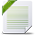# Power BI Exchange

View Only

## Power BI - DAX Formula for IF and count#### Rashmi SiddhalingaiahJul 31, 2019 03:15 PM#### Gopa Kumar SivadasanAug 01, 2019 05:25 AM• #### 1.  Power BI - DAX Formula for IF and count

Posted Jul 31, 2019 03:15 PM
Hi Team ,
I have the data as below  with Class and MD  but i want the result set as Class and Resultset where if a class no contains both FP and MD as their values, it has to represent as ALL else it has to represent the value as such.

 Class No Values Class No Resultset 1 FP 1 All 1 MD 2 All 2 FP 3 MD 2 MD 3 MD

• #### 2.  RE: Power BI - DAX Formula for IF and count

Posted Aug 01, 2019 05:25 AM
Edited by Gopa Kumar Sivadasan Aug 01, 2019 05:26 AM
Hi @Rashmi Siddhalingaiah

Please see if the following helps you.​​

The DAX:

```Result Set =
//Create a distinct table for values column in Table1 for the relevant Class no
VAR rlvntable =
VALUES ( Table1[Values] )
VAR resultset =
IF (
"FP" IN rlvntable
&& "MD" IN rlvntable,
"ALL",
CONCATENATEX ( rlvntable, Table1[Values], "," )
)
RETURN
//avoid calculation if class no is not in scope
IF (
ISINSCOPE ( Table1[Class No] ),
resultset,
BLANK ()
)
```

The above is based on the assumption that the column [values] will have values other than "FP" & "MD". If the [values] column will have only "FP" or "MD", then in the above DAX, the 'CONCATENATEX' part can be replaced by MAX or similar function which returns a scalar value.

PFA the pbix file for your reference.

------------------------------
Gopa Kumar S
------------------------------

Attachment(s)• #### 3.  RE: Power BI - DAX Formula for IF and count

Posted Aug 01, 2019 05:41 AM
Hi Rashmi,

Your Resultsetmeasure should be calculated like this:

```FancyMeasure =
VAR DCount =
DISTINCTCOUNT ( 'Table'[Values] )
VAR DCountAll =
CALCULATE ( DISTINCTCOUNT ( 'Table'[Values] ), ALL ( 'Table' ) )
RETURN
IF (
DCount = DCountAll,
"All",
CONCATENATEX ( 'Table', 'Table'[Values], ", " )
)
​```

------------------------------
Hubert Kobierzewski
Board member
Data Community PL
------------------------------